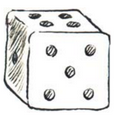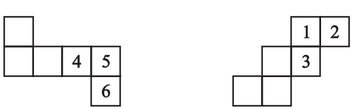Q

# Dice are cubes with dots on each face. Opposite faces of a die always have a total of seven dots on them. Here are two nets to make dice (cubes) the numbers inserted in each square indicate the number of dots in that box.

Q 2     Dice are cubes with dots on each face. Opposite faces of a die always have a total of seven dots on them. Here are two nets to make dice (cubes); the numbers inserted in each square indicate
the number of dots in that box.Insert suitable numbers in the blanks, remembering that the number on the
opposite faces should total to 7.